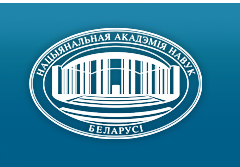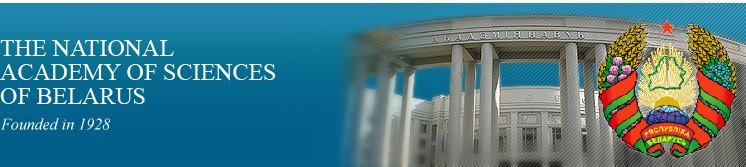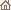Home News About Academy Academy Activities Academy Membership Organizations Publications Conferences Contacts Site Map

# Nonlinear Phenomena in Complex Systems, 1999, Vol.2, No.3/ Publications / Scientific Journals
Nonlinear Phenomena in Complex Systems, 1999, Vol.2, No.3NONLINEAR PHENOMENA IN COMPLEX SYSTEMS An Interdisciplinary Journal Published by The "Education and Upbringing" Publishing Company, Minsk, Republic of Belarus

### Volume 2, Number 3, 1999## CONTENTS

T. Bountis, J.P. Van Der Welle
Subharmonic bifurcations and Melnikov's theory in a system of parametrically driven pendulums. pp. 1--13

Summary: Melnikov's theory of homoclinic bifurcations has proved to be a most successful tool for predicting the onset of (homoclinic) chaos in periodically perturbed dynamical systems of two or more dimensions. By contrast, Melnikov's predictions for the occurrence of subharmonic bifurcations have not been as widely tested and applied to many-dimensional systems of physical significance. In this paper we first demonstrate explicitly the remarkable accuracy of Melnikov's subharmonic theory in predicting the appearance of in-phase and counter-phase oscillations, as well as in-phase rotations, in a system of two damped, parametrically driven pendulums. Secondly, we propose a generalization of Melnikov's approach by which more complicated motions can be studied in coupled pendulum systems of 2N (N2) phase space dimensions.

D. Bainov, E. Minchev
Oscillation of solutions of nonlinear parabolic equations in noncylindrical domains. pp. 14--18

Summary: Forced oscillations of solutions of nonlinear parabolic equations in noncylindrical domains are considered. The case when the force term is zero is investigated separately.

M. V. Bartuccelli
Ladder theorems and positivity of solutions for dissipative partial differential equations. pp. 19--22

Summary: We obtain ladder estimates for semilinear dissipative differential equations. Then we use these estimates to find conditions which assure that solutions with positive initial data are (at least) eventually (i.e. asymptotically) positive. These results are of fundamental importance in many fields of applied mathematics as, for example, biological dynamics, where the solutions of the equations modelling the systems under investigation must often be positive functions.

L.A.Cherkas, V.G.Romanovsky and N.L.Shcheglova
On computing of the limit cycles manifold. pp. 23--27

Summary: An algorithm to compute a non-local analog of Andronov--Hopf manifold for plane polynomial vector fields with a rotating field parameter is described AMS classification scheme numbers.

Margarita Kondrat'eva
Dynamics of quantum averages and quantization conditions in semiclassical approximation. pp. 28--31

Summary: A system of ODE which describes dynamics of quantum averaves of position and momentum operators and second moments in semiclassical approximation is considered (average values are calculated w.r.t. approximate solutions of the evolution Schroedinger equation). It is shown that quantization condition for the Schroedinger operator is related to periodic solutions of the system of ODE. Known semiclassical quantization condition follows from the one obtained here under additional assumptions.

Alexander Rauh
Remarks on unsolved basic problemsof the Navier--Stokes equations. pp. 32--37

Summary: There is renewed interest in the question of whether the Navier--Stokes equations (NSE), one of the fundamental models of classical physics and widely used in engineering applications, are actually self-consistent. After recalling the essential physical assumptions inherent in the NSE, the notion of weak solutions, possible implications for the energy conservation law, as well as existence and uniqueness in the incompressible case are discussed. Emphasis will be placed on the possibility of finite time singularities and their consequences for length scales which should be consistent with the continuum hypothesis.

Krzysztof Sacha, Robert Gebarowski and Jakub Zakrzewski
Classical thresholds for H atom ionization by circularly polarized microwave fields. pp. 38--41

Summary: Ionization of H atoms by circularly polarized microwaves is studied within the classical mechanics framework. We compare recent predictions coming from the Chrikov overlap criterion with results of numerical simulations for finite interaction time. The numerical threshold dependence on the interaction time is shown to obey a power law behaviour for high frequencies.

R. Castro T. D. Sauer
Forecasting and dimension calculations from event timing data. pp. 42--51

Summary: Recent methods for recovering nonlinear dynamical invariants from a sequence of event timings are considered. The analysis is focused on two dynamical invariants: nonlinear forecastability and correlation dimension. The methodology involves geometric reconstruction of the underlying dynamics through the inter-event intervals.

R. M. Yulmetyev, G. P. Vishnevskaya, E. N. Frolova
Manifestation of non-Markovian behavior of molecular system in electron spin relaxation of paramagnetic ions in solutions. pp. 52--60

Summary: Non-Markovian processes and spin memory effects in electron spin relaxation of paramagnetic ions in aqueous, aqua-acid and aqua-glicerol solutions have been investigated. For these purposes the spin-lattice relaxation time of some paramagnetic (Gd3+, Mn2+, Cr3+) ions have been measured by two independent methods: non-resonant paramagnetic absorption method in parallel magnetic fields and EPR. The microscopic mechanism of spin relaxation is the magnetic interparticle dipole-dipole interactions of paramagnetic ions modulated by the translational movement of the particles. Our experimental data for the viscosity dependence of the spin relaxation times exhibited the smooth transition from the liquid-like (diffusional) to the solid-like (rigid-lattice) relaxation scenario in aqueous-acid and aqueous-glicerol solutions. We elaborated the statistical non-Markov theory of paramagnetic relaxation of spins using well-known Zwanzig--Mori's kinetic equations for the normalized spin time correlation functions. Our approach involves the statistical memory effects in combined (spin + solution) system by means of correlation approximation for the second order spin memory function. The theory developed is in good accordance with our experimental results. From our investigations it is obvious that non-Markov processes and spin memory effects play a crucial role in the determination of the character of paramagnetic relaxation of electron spins in solutions.

L. M. Barkovsky, A. N. Furs
Tensor eikonal approximations in asymptotic expansions for stratified media in the absence of commutation. pp. 61--71

Summary: The tensor eikonal method for solving wave equations of optics of stratified anisotropic media is proposed. Geometro-optical solutions of these equations are presented in the covariant three-dimensional evolutional form. Such representation allows to operate with electromagnetic fields in coordinate free representation without any expansion of the fields into partial waves. The eikonal tensors determined by wave polarization (photon spin) are involved in the evolution Cauchy operators and are expressed in terms of the normal refraction tensors. These tensors are non-commutative in the general case and necessity of disentangling the evolution operators arises even if a medium is isotropic. With the use of standard operator procedures such disentangling is carried out for oblique incidence of polarized monochromatic light to isotropic layered media. For this case the closed expressions describing the spatial evolution of fields are obtained.

A.P. Blokhin, M.F. Gelin, and A.V. Uvarov
Orientational relaxation of macromolecules immobilized on a surface. pp. 72--81

Summary: To investigate the influence of inertial effects on the orientational relaxation of macromolecules subjected to rigid intersubunit constraints and placed in a buffer medium, the diffusion equation with memory (DEM) is deduced from the Fokker-Planck equation (FPE) in case of arbitrary generalized coordinates (the FPE has been derived by us elsewhere [1,2]). The DEM allows us to establish a correct short time behavior for correlation functions (CFs) of generalized coordinates. The DEM is invoked to perform illustrative calculations of the orientational CFs for immobilized on a surface once broken nonrigid rod in two dimensions (2D). These calculations reveal that the CFs under certain conditions exhibit an oscillatory behavior, which is irreproducible within the standard diffusion equation (DE). Several methods are considered for the approximate solution of the DEM, and their application to three dimensional (3D) DEMs is discussed.

L.F. Babichev, D.V. Klenitsky, V.I. Kuvshinov
Fractal structure in the first order QCD phase transition. pp. 82--85

Summary: Dynamical fluctuations, induced by the QCD first order phase transition in nuclear collisions, are studied. Their fractal structure is discussed.

N.I. Stetyukevich, O.P. Kuznechik, V.N. Gorenkov
IR-emission characteristics of crystalline hydrates in the processes of melting and crystallisation. pp. 86--90

Summary: The influence of self-organization of molecular structure on emission characteristics of crystalline hydrates 2-methyl-2-propanols and sodium thiosulphate in the range of 4000--400 cm-1 has been investigated. The surface flux density of thermal emission in the processes of crystallisation and melting has been measured. Possible mechanisms of generation of non-equilibrium emission has been discussed. The obtained results may be used for remote control of the phase transitions.

A. S Dmitriev, A. I. Panas, L.V.Kuzmin
Chaotic synchronization and chaotic communications over a band-pass channel. pp. 91--99

Summary: We propose a method for combating the transmitted signal filtering in the communication channel, based on a special design of chaotic oscillator. In the method, an element that has the same filtering properties as the channel is added to the feedback loop of the chaotic source. It gives possibility to obtain perfect chaotic synchronous response in the receiver. The method is illustrated by numerical simulation and physical experiments.

V.I. Kuvshinov, A.V.Kuzmin
Chaos-order transitions in SU(2) Yang--Mills--Higgs systems. pp. 100--105

Summary: In this work we briefly discuss the problem of chaos in classical gauge field theories. It is not a full consideration of such questions. Rather it is an introduction. We give a qualitative description of the methods widely used to study the chaotic behaviour of the classical field systems and discuss some investigations of these questions.

Designed and maintained by Dr. Nikolai N. Kostyukovich. Last updated: January 11, 2000
Created with assistance of Dr. Leonid F. Babichev
Copyright © 2000 The National Academy of Sciences of Belarus
Copyright © 1999 The Nonlinear Phenomena in Complex Systems